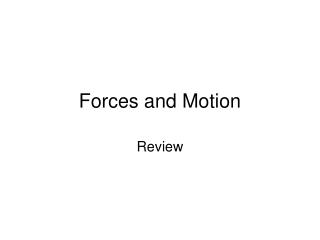# Forces and Motion - PowerPoint PPT PresentationDownload PresentationForces and Motion

Forces and Motion
Download Presentation## Forces and Motion

- - - - - - - - - - - - - - - - - - - - - - - - - - - E N D - - - - - - - - - - - - - - - - - - - - - - - - - - -
##### Presentation Transcript

1. Forces and Motion Review

2. Which object as a periodic motion? A. a car B. the Earth C. a sea star D. a pendulum

3. Which object as a periodic motion? A. a car B. the Earth C. a sea star D. a pendulum

4. A train travels 140 miles west in 2 hours. What is the velocity of the train? A. 70 miles per hour B. 280 miles per hour C. 70 miles per hour/west D. 280 miles per hour/west

5. A train travels 140 miles west in 2 hours. What is the velocity of the train? A. 70 miles per hour B. 280 miles per hour C. 70 miles per hour/west D. 280 miles per hour/west

6. How do electricity and magnetism act on different objects? A. They can only pull objects. B. The can only push objects. C. They can either push or pull objects. D. Than can either push or pull objects only if they touch

7. How do electricity and magnetism act on different objects? A. They can only pull objects. B. The can only push objects. C. They can either push or pull objects. D. Than can either push or pull objects only if they touch

8. Machine A performs 48 joules of work in 4 seconds. Machine B performs the same amount of work in half the time. Which statement is true about Machines A and B? A. Machines A and B used no power. B. Machine A used more power than Machine B. C. Machine B used more power than Machine A. D. Machine A and Machine B used the same amount of power.

9. Machine A performs 48 joules of work in 4 seconds. Machine B performs the same amount of work in half the time. Which statement is true about Machines A and B? A. Machines A and B used no power. B. Machine A used more power than Machine B. C. Machine B used more power than Machine A. D. Machine A and Machine B used the same amount of power.

10. When you ride a roller coaster, what makes your body rise out of its seat at the top of a hill? A. inertia B. gravity C. friction D. electricity

11. When you ride a roller coaster, what makes your body rise out of its seat at the top of a hill? A. inertia B. gravity C. friction D. electricity

12. How can you complete this sentence so it is always true? The greater the net force acting on an object . . . A. the less the mass of an object. B. the greater the mass of an object C. the less the acceleration of the object. D. the greater the acceleration of the object.

13. How can you complete this sentence so it is always true? The greater the net force acting on an object . . . A. the less the mass of an object. B. the greater the mass of an object C. the less the acceleration of the object. D. the greater the acceleration of the object.

14. A truck hits an insect. What effect does the insect have on the truck? A. The insect has no effect on the truck. B. The insect hits the truck and the truck hits the insect with the same amount of force. C. The insect hits the truck with less force than the force with which the truck hits the insect. D. The insect hits the tuck with more force than the force with which the truck hits the insect.

15. A truck hits an insect. What effect does the insect have on the truck? A. The insect has no effect on the truck. B. The insect hits the truck and the truck hits the insect with the same amount of force. C. The insect hits the truck with less force than the force with which the truck hits the insect. D. The insect hits the tuck with more force than the force with which the truck hits the insect.

16. Which force keeps a satellite in orbit? A. gravity B. friction C. electricity D. magnetism

17. Which force keeps a satellite in orbit? A. gravity B. friction C. electricity D. magnetism

18. A student says that if she throws a ball in space it will not slow down as quickly as it would be on Earth. Is the student correct?

19. A student says that if she throws a ball in space it will not slow down as quickly as it would be on Earth. Is the student correct? Yes! Because in space there is less friction to resist the ball’s motion than there is on Earth. This means that the ball will continue to move more easily than it would on Earth.